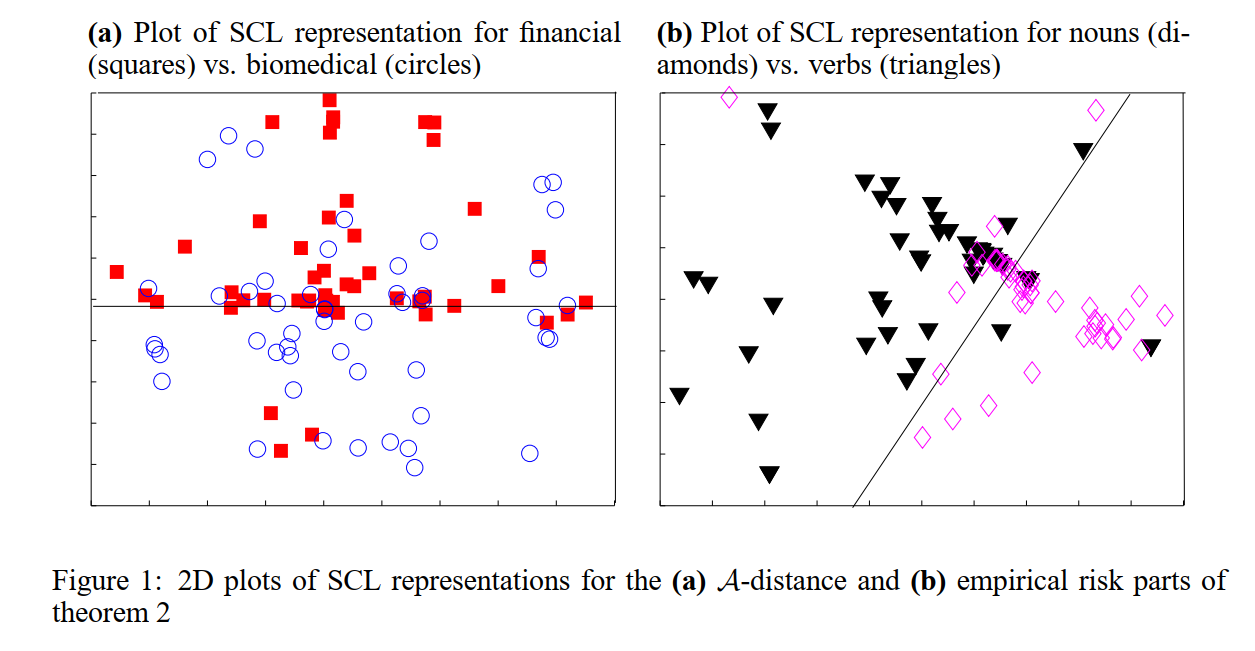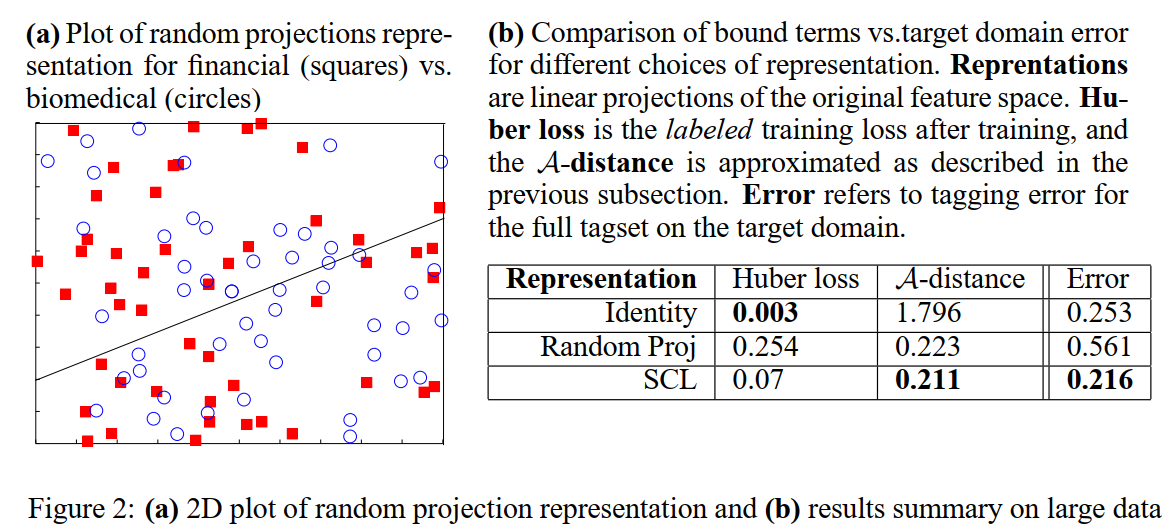## 0. 相关符号含义

$\chi$：样本实例集合

$\cal Z$：特征空间

$\cal D$：在$\chi$上的一个分布

$f$：$\chi \to [0, 1]$，$f(x)$代表$x$的标签是1的概率

$\cal R$：表示函数，将实例映射到特征$\cal R: \cal X \to \cal Z$

${\cal D}_S$：源域样本分布

${\cal \tilde{D}}_S$：源域样本在特征空间$\cal Z$上的感应（induced）分布

${\cal D}_T$：目标域样本分布

${\cal \tilde{D}}_T$：目标域样本在特征空间$\cal Z$上的感应分布

$h$：预测函数，从特征空间$\cal Z$到$[0, 1]$

$\epsilon_S(h)$：$h$关于$\cal D_T$的期望误差

${\rm sup}$：上确界

${\rm inf}$：下确界

## 1. 一般学习问题与DA的形式化

### 1.2 DA问题的定义

“域”的含义是实例集$\cal X$的分布$\cal D$。我们假设有两个域，源域（source domain）目标域（target domain）。${\cal D}_S$是源域样本分布，${\cal \tilde{D}}_S$是源域样本在特征空间$\cal Z$上的感应分布。同样的含义，我们同${\cal D}_T$和${\cal \tilde{D}}_T$表示目标域。$f: {\cal X} \to [0, 1]$是标记规则函数，在源域和目标域上都适用，$\tilde{\cal f}$是$f$在$\cal R$下的感应。

## 2. DA的泛化边界

DA的各种方法适用带标签的源域数据训练分类器，它会在目标域数据集上有泛化误差。这个泛化误差包括两项：

• 分类器在源域数据集上的误差边界
• 感应的源域边缘分布${\cal D}_S$和感应的目标域边缘分布${\cal D}_T$之间的差异性

$$d_{\mathcal{A}}\left(\mathcal{D}, \mathcal{D}^{\prime}\right)=2 \sup _{A \in \mathcal{A}}\left|\operatorname{Pr}_{\mathcal{D}}[A]-\operatorname{Pr}_{\mathcal{D}^{\prime}}[A]\right|$$

###### Theorem 1：

${\cal R}$是从$\cal X$到$\cal Z$的表示函数，$\cal H$是VC维为$d$的假设类空间，如果$m$条随机样本是从应用了${\cal R}$函数的${\cal D}_S$分布上独立同分布地采样得到，其标签由$f$标记，那么对于每个$h \in {\cal H}$，下式至少以$1-\delta$的概率成立：

###### Theorem 2：

${\cal R}$是从$\cal X$到$\cal Z$的表示函数，$\cal H$是VC维为$d$的假设类空间，如果$m$条随机样本是从应用了${\cal R}$函数的${\cal D}_S$分布上独立同分布地采样得到，其标签由$f$标记；并且${\tilde {\cal U}_S}$和${\tilde {\cal U}_T}$是从${\tilde {\cal D}_S}$和${\tilde {\cal D}_T}$上采样得到的无标签样本，都分别有$m^{\prime}$个，那么对于每个$h \in {\cal H}$，下式至少以$1-\delta$的概率成立：

$$\epsilon_{T}(h) \leq \hat{\epsilon}_{S}(h)+\frac{4}{m} \sqrt{\left(d \log \frac{2 e m}{d}+\log \frac{4}{\delta}\right)}+\lambda+d_{\mathcal{H}}\left(\tilde{\mathcal{U}}_{S}, \tilde{\mathcal{U}}_{T}\right)+4 \sqrt{\frac{d \log \left(2 m^{\prime}\right)+\log \left(\frac{4}{\delta}\right)}{m^{\prime}}}$$

## 3. $\cal A$-距离的计算

$$\operatorname{err}(\mathrm{h})=\frac{1}{2 \mathrm{m}^{\prime}} \sum_{\mathrm{i}=1}^{2 \mathrm{m}^{\prime}}\left|\mathrm{h}\left(\mathbf{z}_{\mathrm{i}}\right)-\mathrm{I}_{\mathrm{z}_{\mathrm{i}} \in \tilde{\mathcal{U}}_{\mathrm{S}}}\right|$$

$$d_{A}\left(\tilde{\mathcal{U}}_{S}, \tilde{\mathcal{U}}_{T}\right)=2\left(1-2 \min _{h^{\prime} \in \mathcal{H}} \operatorname{err}\left(\mathrm{h}^{\prime}\right)\right)$$

## 4. 实验